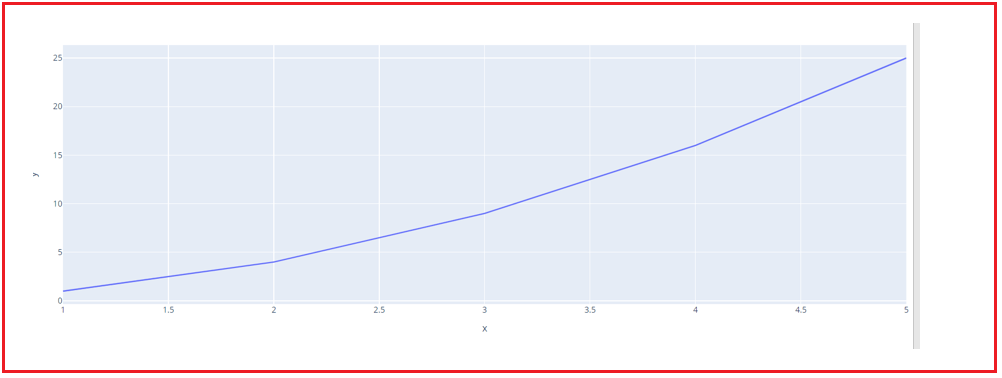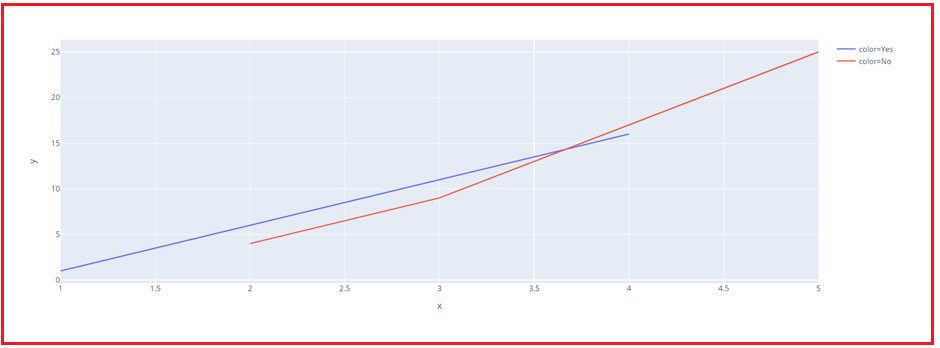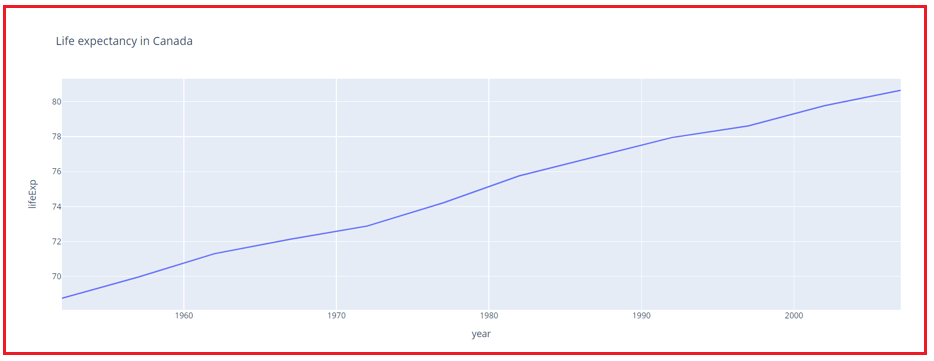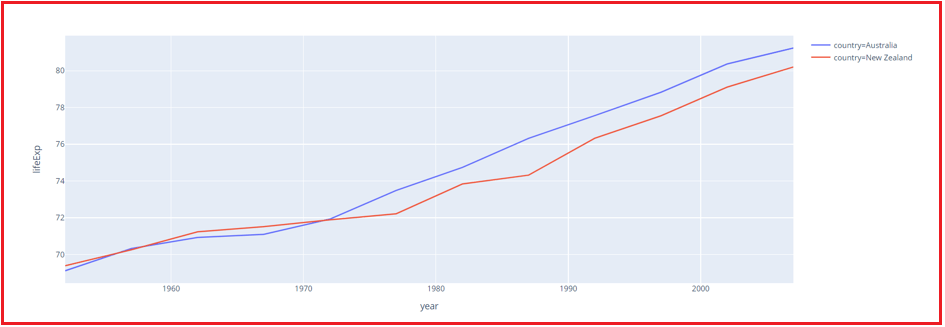# Line Charts in Python using Plotly

## Line Charts in Python using Plotly

In this article, I am going to discuss Line Charts in Python using Plotly for Data Science with Examples. Please read our previous article where we gave a brief introduction to Plotly for Data Science.

##### Line Charts in Python using Plotly

A line chart is a visual representation of data that changes over time. A line chart is made by drawing a set of points and connecting them with a straight line. Line charts are used to track changes through time, both short and long. Let’s have a look at some examples of Line Charts using Plotly.

###### Example 1 – Creating Line Chart for data points
```# Creating a basic Line Plot
import plotly.express as px

xd = [1,2,3,4,5]
yd = [1,4,9,16,25]

fig = px.line(x=xd, y=yd)
fig.show()
```
###### Output:###### Example 2 – Creating Line Chart for data points for different categories
```# Creating a Line Plot with Encoding Color for a Category
import plotly.express as px

xd = [1,2,3,4,5]
yd = [1,4,9,16,25]
zd = ['Yes', 'No', 'No', 'yes', 'No']

fig = px.line(x=xd, y=yd, color=zd)
fig.show()
```
###### Output:###### Example 3 – Creating a line chart for a dataset
```import plotly.express as px

fig = px.line(df, x='year', y='lifeExp', title='Life Expectancy in Canada')
fig.show()
```
###### Output:###### Example 4 – Creating Line Chart for data points for different categories
```import plotly.express as px

df = px.data.gapminder().query("continent=='Oceania'")
fig = px.line(df, x='year', y='lifeExp', color='country')
fig.show()
```
###### Output:In the next article, I am going to discuss Scatter and Bubble Plots in Python using Plotly for Data Science with Examples. Here, in this article, I try to explain Line Charts in Python using Plotly for Data Science with Examples. I hope you enjoy this Line Charts in Python using Plotly for Data Science with Examples article.